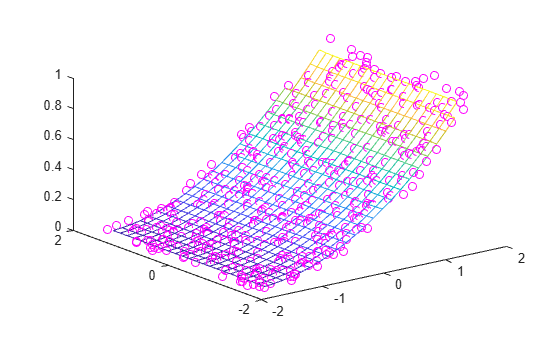# Normalize Data with Differing Magnitudes

This example shows how to use normalization to improve scattered data interpolation results with `griddata`. Normalization can improve the interpolation results in some cases, but in others it can compromise the accuracy of the solution. Whether to use normalization is a judgment made based on the nature of the data being interpolated.

• Benefits: Normalizing your data can potentially improve the interpolation result when the independent variables have different units and substantially different scales. In this case, scaling the inputs to have similar magnitudes might improve the numerical aspects of the interpolation. An example where normalization would be beneficial is if `x` represents engine speed in RPMs from 500 to 3500, and `y` represents engine load from 0 to 1. The scales of `x` and `y` differ by a few orders of magnitude, and they have different units.

• Cautions: Use caution when normalizing your data if the independent variables have the same units, even if the scales of the variables are different. With data of the same units, normalization distorts the solution by adding a directional bias, which affects the underlying triangulation and ultimately compromises the accuracy of the interpolation. An example where normalization is erroneous is if both `x` and `y` represent locations and have units of meters. Scaling `x` and `y` unequally is not recommended because 10 m due East should be spatially the same as 10 m due North.

Create some sample data where the values in `y` are a few orders of magnitude larger than those in `x`. Assume that `x` and `y` have different units.

```x = rand(1,500)/100; y = 2.*(rand(1,500)-0.5).*90; z = (x.*1e2).^2; ```

Use the sample data to construct a grid of query points. Interpolate the sample data on the grid and plot the results.

```X = linspace(min(x),max(x),25); Y = linspace(min(y),max(y),25); [xq, yq] = meshgrid(X,Y); zq = griddata(x,y,z,xq,yq); plot3(x,y,z,'mo') hold on mesh(xq,yq,zq) xlabel('x') ylabel('y') hold off```The result produced by `griddata` is not very smooth and seems to be noisy. The differing scales in the independent variables contribute to this, since a small change in the size of one variable can lead to a much larger change in the size of the other variable.

Since `x` and `y` have different units, normalizing them so that they have similar magnitudes should help produce better results. Normalize the sample points using z-scores and regenerate the interpolation using `griddata`.

```% Normalize Sample Points x = normalize(x); y = normalize(y); % Regenerate Grid X = linspace(min(x),max(x),25); Y = linspace(min(y),max(y),25); [xq, yq] = meshgrid(X,Y); % Interpolate and Plot zq = griddata(x,y,z,xq,yq); plot3(x,y,z,'mo') hold on mesh(xq,yq,zq)```In this case, normalizing the sample points permits `griddata` to compute a smoother solution.